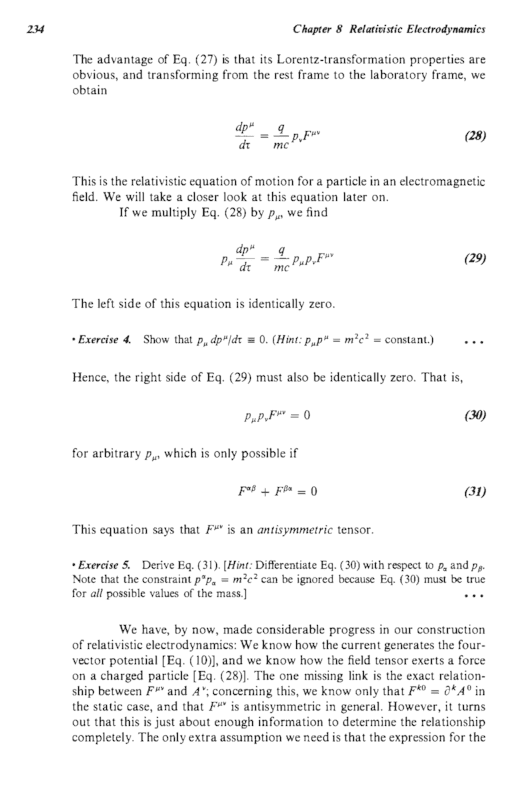# Antisymmetry of the electromagnetic field tensor

shinobi20
Homework Statement:
Show that the electromagnetic field tensor is antisymmetric from the fact that ##p_\mu p_\nu F^{\mu \nu} = 0##.
Relevant Equations:
##p_\mu p_\nu F^{\mu \nu} = 0##
where ##p_\mu## is the 4-momentum and ##F^{\mu \nu}## is the EM field tensorI am trying to answer exercise 5 but I am not sure I understand what the hint is implying, differentiate with respect to ##p_\alpha## and ##p_\beta##, I have done this but nothing is clicking. Also, what is the relevance of the hint "the constraint ##p^\alpha p_\alpha = m^2c^2## can be ignored ..."? Please help me clarify what the author wants me to think or do in accordance to the hint.

•JD_PM

mitochan
##p_\mu p_\nu## is a symmetric tensor.

Gold Member
try writing $F^{\mu\nu}$ as a sum of symmetric and anti symmetric pieces then do the contraction with the $p^\alpha$ to show that the components cancel.

or try writing $F^{\mu\nu}$ using its definition in terms of $A^\mu$ and $\partial^\nu$

shinobi20
try writing $F^{\mu\nu}$ as a sum of symmetric and anti symmetric pieces then do the contraction with the $p^\alpha$ to show that the components cancel.

or try writing $F^{\mu\nu}$ using its definition in terms of $A^\mu$ and $\partial^\nu$

I cannot figure out what you want me to do, but then I want to follow the hint given in OP.

My attempt, differentiating with respect to ##p_\alpha## and ##p_\beta##,

##\delta^\mu_\alpha p_\nu F^{\mu\nu} + p_\mu \delta^\nu_\alpha F^{\mu\nu} = 0 \quad \rightarrow \quad p_\nu F^{\alpha\nu} + p_\mu F^{\mu\alpha} = 0##

##\delta^\mu_\beta p_\nu F^{\mu\nu} + p_\mu \delta^\nu_\beta F^{\mu\nu} = 0 \quad \rightarrow \quad p_\nu F^{\beta\nu} + p_\mu F^{\mu\beta} = 0##

Multiplying both sides of the first equation by ##p^\mu##

##p^\mu p_\nu F^{\alpha\nu} + m^2 c^2 F^{\mu\alpha} = 0##

Multiplying both sides of the second equation by ##p^\nu##

##m^2 c^2 F^{\beta\nu} + p^\nu p_\mu F^{\mu\beta} = 0##

From these last two equations, I think there are only a few steps before I get the answer, but I cannot see it.

**I may be wrong by multiplying ##p^\nu## in the second equation, maybe it should also be ##p^\mu##, but the flow is as above.

mitochan
(Further to post #2)
$$p_\mu p_\nu F^{\mu \nu}=p_\mu p_\nu (S^{\mu \nu}+A^{\mu \nu})$$
where S is symmetric and A is anti-symmetric components of F.
Contract of symmetric tensor, here ##p_\mu p_\nu##, and anti-symmetric tensor, here ##A^{\mu \nu}##, is always zero.
$$p_\mu p_\nu A^{\mu \nu}=0$$
This and
$$p_\mu p_\nu F^{\mu \nu}=0$$
give
$$p_\mu p_\nu S^{\mu \nu}=0$$
$$S^{\mu \nu}=0$$
So ##F^{\mu \nu}## has only anti-symmetric components.

Staff Emeritus
Homework Helper
I cannot figure out what you want me to do, but then I want to follow the hint given in OP.

My attempt, differentiating with respect to ##p_\alpha## and ##p_\beta##,

##\delta^\mu_\alpha p_\nu F^{\mu\nu} + p_\mu \delta^\nu_\alpha F^{\mu\nu} = 0 \quad \rightarrow \quad p_\nu F^{\alpha\nu} + p_\mu F^{\mu\alpha} = 0##
Try differentiating this result with respect to ##p_\beta##.

shinobi20
Try differentiating this result with respect to ##p_\beta##.
😑😑😑
I don't consider myself stupid, but there are some days where I think I am one. It is like having a snake in front of me but still don't notice it. The hint given in OP is very clear, you just restated what it said but in a different way.

What is your advice on this? Is this just a physics thing that can be improved within physics?Last edited:
Staff Emeritus
What is your advice on this? Is this just a physics thing that can be improved within physics?•